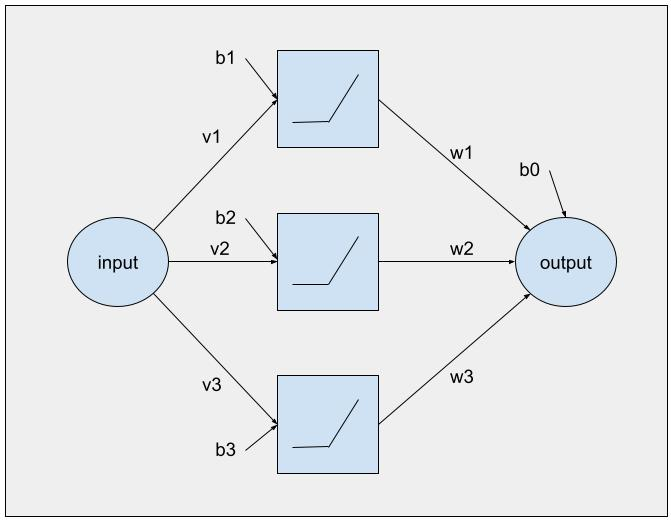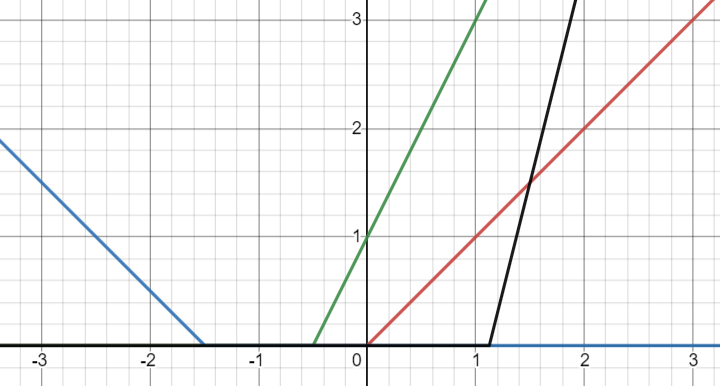# Let’s manually approximate a simple function with a ReLU neural network

I was playing around with a homemade implementation of a neural network with Rectified Linear Units as the basic building blocks. I’d read that a network with a single hidden layer of these should in principle be capable of approximating any continuous 1-dimensional function, but had some trouble intuitively grasping why that should be true. The underlying principle is that we generate a piecewise-linear approximation of whatever the real function is, which is clear enough. But what happens with the input values as they flow through the network?

Let’s start at the beginning: the ReLU activation function is simply this:

f(x) = max(0, x)

It’s just the input value if that is greater or equal 0, or otherwise 0.

We can use these units in a neural network, in this case we have 1 input neuron, 3 ReLUs in the hidden layer and 1 output neuron:Neural network with 1 hidden layer

Each ReLU has one bias value and one weight (b and v values) so the output of the entire unit in respect to its single input x is:

f(x) = max(0, b + v*x)

The output neuron accumulates the weighted outputs of each ReLU. It is itself NOT a ReLU, or we could only generate functions that have no negative values! The output z is then, in respect to ReLU output values r1, r2, r3:

z=b0 + w1*r1 + w2*r2 + w3*r3

## What comes out of each ReLU in isolation?

Given a bias and a weight value, we can visualize that each ReLU in the hidden layer generates a “ray” starting somewhere on the x-axis which radiates in some (always upward!) direction, and anywhere else is 0. Some examples of ReLU outputs:Possible outputs of each ReLU (thanks to Desmos for the online function plotter)

The output neuron will multiply each of these inputs with a certain value, which can be interpreted as scaling them vertically (or inverting them if the weight is negative). All the scaled ReLUs are combined by adding them up, and then the output’s bias moves the whole thing up or down vertically.

## Building a piecewise-linear function

Let’s say we have been given a function to approximate which is defined by 3 line segments (we only care about the input range -1 ≤ x ≤ 1) between these 4 points:

The graph looks like this:

I would like to use each ReLU to generate one line segment each, starting with the leftmost segment being generated by ReLU 1, and then each following segment being generated by the next ReLU. With 3 segments we should need 3 ReLUs.

I found this trick to simplify the construction: we can hold the weights of the ReLUs at 1, and simply adjust their bias to move the generated “ray” left and right until the starting point is the starting x value of the segment the ReLU is supposed to generate. Then we adjust the relevant output weight so we get the desired direction of the ray. What this means is that each ReLU “bends” the previous ReLU’s ray into the direction we need for the new segment (because we effectively add one linear function to another, yielding a different linear function). So we start with these rays as the outputs of each ReLU, giving us the bias values b1, b2, b3 (=1, 0.5, 0) and all ReLU weights v1=v2=v3=0:

The first ReLU generates the first line segment, for which we will need to set the ray direction (requiring the associated output weight w1=4). We also need to set the output bias so that it starts at the correct y-axis position (b0=-1):

Now we hold the known values and adjust the output weight for ReLU 2 to generate segment 2 (yielding w2=-8):

And finally we do segment 3 with ReLU 3 (yielding w3=5 for the output weight):

So in principle using this approach we should be able to generate any piecewise linear (1-dimensional) function of N segments with a network that has N ReLUs in the hidden layer.

Thinking about what this means for generating the network’s parameters with e.g. a stochastic algorithm, it’s clear that depending on the ReLU “rays” setup twiddling some parameters will have a greater impact on the entire function than others. For example, in the completed network changing the weight associated with ReLU 1 also affects everything to the right of segment 1: